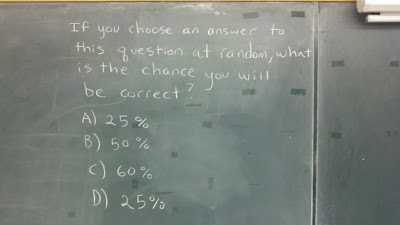## Friday, October 28, 2011

### My Brain HurtsWell, if I choose any answer at random and there are only four to choose from, then I must have a 25% chance of being correct. Yet two of the four answers are 25% so I must have a 50% chance of selecting the correct answer.

But wait. The 60% answer can never be correct because that would mean I had to choose more than one answer. So that's at least 25% that I would be incorrect. And since I have a 25% chance of selecting a single correct answer and a 50% chance of selecting two of four correct answers, I really have a 75% chance of being correct. But 75% is not one of the answers so that is incorrect.

But wait. If I pick 50%, I'm incorrect because there's only a 25% chance of choosing 50%. And I'm incorrect if I pick 25% because there is a 50% chance I could choose 25%. And 60% is incorrect because I'd have to pick more than one answer which is not allowed. So the real answer is 0%, which is wrong because it's not one of the answers.

But wait. Since there's one answer with 50%, I have a 25% chance of randomly selecting the correct answer of 50% which would be incorrect because I have a 25% chance of picking 50% which, in turn, is incorrect because I have a 50% chance of picking 25%. And that would be incorrect because there's only a 25% chance of picking 50% which would again be incorrect....

Thank goodness no Sicilians or iocane powder are involved.

#### 1 comment:Anonymous said...

42